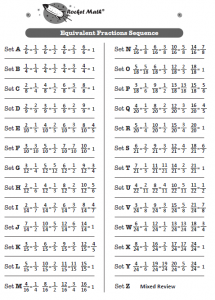## Why commit to memory equivalent fractions?

We expect students to reduce their answers to the lowest terms, but how can they if they don’t recognize them?  To do this easily, students need to know that six-eighths is equivalent to three-fourths and that four-twelfths is equivalent to one-third.  While they can calculate these, it is very helpful to know the most common equivalent fractions by memory.  Not knowing these by memory accounts for the most common problem students have in fractions: failing to “reduce their answers to simplest form.”

Each set (A through Z) has four fractions which are displayed on a fraction number line.  In addition to pairs of equivalent fractions, students frequently learn fractions equivalent to one, such as ten-tenths.  They also memorize fractions that can’t be reduced, for example three-fourths is only equivalent to three-fourths. Students use a number line and identify equivalent fractions by circling an equivalent pair of fractions.  They understand why they are equivalent as well as memorizing the pair.

Equivalent fractions, Factors, and Integers, are all pre-algebra programs that are recommended for upper elementary and middle school students who already know the basic facts.

## 5-minute Equivalent Fractions video lesson.

Dr. Don explains How the Equivalent Fractions program works.

## Students will commit to memory 100 common equivalent fractions.

Click here for the full sequence of equivalent fractions that students will learn in this program.